# 2018年天津小升初数学模块练习题：四则混合计算2018年天津小升初数学模块练习题：四则混合计算

一、填空：

1、根据2516÷68=37，直接写出下列各题得数：

251.6÷6.8=( ) 25.16÷0.37=( ) 0.068×3.7=( )

2、在( )内填入适当的运算符号或数据：

1)0.43( )1000=430 2.46×( )=24.6 12.5( )100=0.125 0.03×( )=30

2)( )×0.3×8.54=0 64×125=( )×8×125

4.375-(1 + )=( )-1 3.87×18-38.7×0.8=( )×(18-8)

( )×0.78+0.22×( )=36.4×(0.78+0.22) 63.63÷( )÷0.9=6363÷63

3) ×( )=2 ( )÷3 =2 1 ×( )=( )×1 =1 ×( )

( )÷ = ÷( )=( )÷1

6 ×( )=6 ÷( ) 4 ×( )=( )÷4

3、 的分数单位与0.7的小数单位之和是( )。

4、比较□两边的算式，选择一个合适的符号(>、<、=)填在 内：

1)3.4×2.5□3.4 9 ÷1.2□9 × □ 0.1×10□0.1÷0.1

8 ÷ □( +8)×1.25 14 ×15□14×15+ ÷

( +2.4)×0.25□ +2.4×0.25 8 +1.66- □8.8+1 -0.375

5、两数相除，商3余4，如果把被除数、除数、商及余数相加，和是43，被除数是( )，除数是( )。

6、有一个整数与它自己相加、相减、相乘、相除，把所得的和、差、积、商加起来等于36，这个数是( )。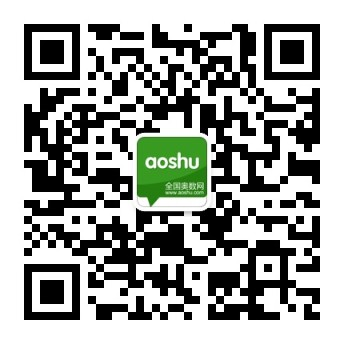您还可以通过手机、平板电脑等移动设备访问天津奥数网，升学路上有我们相伴。>>[点击查看]

•欢迎扫描二维码
关注奥数网微信
ID：aoshu_2003

•欢迎扫描二维码
关注中考网微信
ID：zhongkao_com

### 小学新闻导航

1.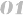北京小学新闻 上海小学新闻 广州小学新闻 深圳小学新闻
2.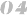天津小学新闻 武汉小学新闻 成都小学新闻 石家庄小学新闻
3.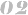南京小学新闻 杭州小学新闻 济南小学新闻 苏州小学新闻
4.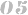郑州小学新闻 沈阳小学新闻 太原小学新闻 重庆小学新闻
5.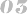长沙小学新闻 合肥小学新闻 宁波小学新闻 青岛小学新闻